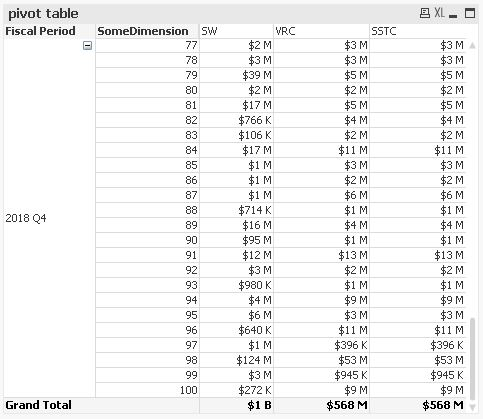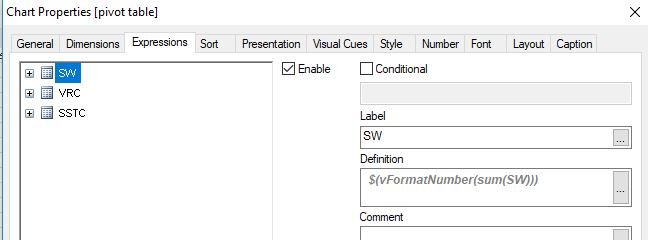# QlikView App Dev

Discussion Board for collaboration related to QlikView App Development.

Announcements
Join this live chat April 6, 10AM EST - QlikView to Qlik Sense REGISTER
cancel
Showing results for
Search instead for
Did you mean:Master

## pivot table with dollor and M

Hi All,

i want to display the result as shown in image

output:  Pivot table

dimension :FiscalPeriod

Measure:

sum(SW)

sum(VRC)

sum(SSTC)

6 RepliesPartner

Hi Sony,

did you try to apply the solution reported here Re: Number formatting - millions to billions or this one Format Number (in Thousand) ?

Let us know.

SimoneMaster
Author

thnks  it  is working it seems  but if i want   "K"  how can i include in this expression?

in below expression only B and M is there i want to include K also how to do this?

=If(sum(Amount)/1000000000 > 1,Num(sum(Amount)/1000000000,'\$#,###B'), Num(sum(Amount)/1000000,'\$#,###M'))Master
Author

and in this i dont want  comma    how i can remove comma and   include \$ symbol

how can i do this?Partner

Hi,

please try this:

=If(sum(SW)/1000000000 > 1,Num(round(sum(SW)/1000000000),'\$ ####B'), If(sum(SW)/1000000>1,Num(round(sum(SW)/1000000),'\$ ####M'), Num(round(sum(SW)/1000),'\$ ####K')))

Let me know 🙂

SimoneMaster
Author

thanks sir its working in my sample app.

i need to test in real data and then i will close this one.MVP

Hi,

one example using your sample data:```SET vSize = Div(Log10(\$1),3);
SET vFormatNumber = Dual(Num(\$1/Pow(10,3*\$(vSize(\$1))),'\$#,##0')&' '&Pick(\$(vSize(\$1)),'K','M','B','T'),\$1);

table1:
LOAD [Fiscal Period],
Ceil(Rand()*100) as SomeDimension,
SW,
VRC,
[Grand Total],
SSTC,
HHTC
FROM [https://community.qlik.com/servlet/JiveServlet/download/1538862-336899/Dim_Test.xlsx] (ooxml, embedded labels, table is Sheet2);
```

hope this helps

regards

Marco